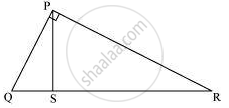# Prove that in a right triangle, the square of the hypotenuse is equal to the sum of the squares of the other two sides. - Mathematics

Sum

Prove that in a right triangle, the square of the hypotenuse is equal to the sum of the squares of the other two sides.

#### Solution

To prove: In a right-angled triangle, the square of the hypotenuse is equal to the sum of squares of the other two sides of the triangle.

Proof: Let PQR be a triangle, right-angled at P.
Draw PS ⊥ QRNow, we know that if a perpendicular is drawn from the vertex of the right angle of a right triangle to the hypotenuse, then the triangles on both sides of the perpendicular are similar to the whole triangle and to each other.

∴ΔQSP ∼ ΔQPR

Therefore, "QS"/"QP" = "QP"/"QR" .....................(Since the sides of similar triangles are proportional)

⇒ QS. QR = Qp2 .......(1)

Also, we have
ΔPSR ∼ ΔQPR

Therefore, "RS"/"RP" = "RP"/"RQ".............(Since the sides of similar triangles are proportional)

⇒ RS.RQ = RP2 … (2)

Adding equations (1) and (2), we obtain

QS.QR + RS.RQ = RP2 + QP2

⇒ QR. (QS + RS) = RP2 + QP2

⇒ QR.QR = RP2 + QP2

⇒ QR2 = RP2 + QP2

Thus, the square of the hypotenuse is equal to the sum of squares of the other two sides of the triangle.

Concept: Areas of Similar Triangles
Is there an error in this question or solution?